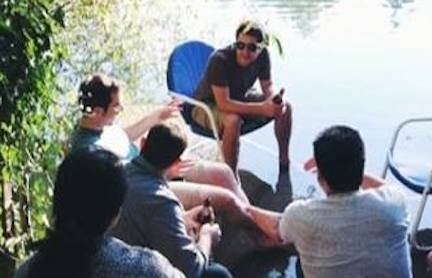# Let's Play A Game

Probability Level 4I pick a subset $S$ of {Sue, Arron, Pete, Dan, Calvin}.

You make a guess of any subset $T$, and I will then tell you how many elements there are in $T \cap S$.

You continue making different choices of $T$ until you are able to exactly determine $S$. What is the minimum number of guesses that are necessary?

Note: You do not need to pick $S$ as one of your choices.

###### Image credit: Cece Yu
×

Problem Loading...

Note Loading...

Set Loading...